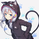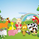# Parametric Corrective Linear Moving Averages

‎1799‎ مشاهدة
Impulse responses can fully describe their associated systems, for example a linearly weighted moving average ( WMA ) has a linearly decaying impulse response, therefore we can deduce that lag is reduced since recent values are the ones with the most weights, the Blackman moving average (or Blackman filter) has a bell shaped impulse response, that is mid term values are the ones with the most weights, we can deduce that such moving average is pretty smooth, the least squares moving average has negative weights, we can therefore deduce that it aim to heavily reduce lag, and so on. We could even estimate the lag of a moving average by looking at its impulse response (calculating the lag of a moving average is the aim of my next article with Pinescripters).

Today a new moving average is presented, such moving average use a parametric rectified linear unit function as weighting function, we will see that such moving average can be used as a low lag moving average as well as a signal moving average, thus creating a moving average crossover system. Finally we will estimate the LSMA using the proposed moving average.

Correctivity And The Parametric Rectified Linear Unit Function

Lot of terms are used, each representing one thing, lets start with the easiest one,"corrective". In some of my posts i may have used the term "underweighting", which refer to the process of attributing negative weights to the input of a moving average, a corrective moving average is simply a moving average underweighting oldest values of the input, simply put most of the low lag moving averages you'll find are corrective. This term was used by Aistis Raudys in its paper "Optimal Negative Weight Moving Average for Stock Price Series Smoothing" and i felt like it was a more elegant term to use instead of "low-lag".

Now we will describe the parametric rectified linear unit function (PReLU), this function is the one used as weighting function and is not that complex. This function has two inputs, alpha, and x, in short if x is greater than 0, x remain unchanged, however if x is lower than 0, then the function output is alpha × x, if alpha is equal to 1 then the function is equivalent to an identity function, if alpha is equal to 0 then the function is equivalent to a rectified unit function.

PReLU is mostly used in neural network design as an activation function, i wont explain to you how neural networks works but remember that neural networks aim to mimic the neural networks in the brain, and the activation function mimic the process of neuron firing. Its a super interesting topic because activation functions regroup many functions that can be used for technical indicators, one example being the inverse fisher RSI who make use of the hyperbolic tangent function.

Finally the term parametric used here refer to the ability of the user to change the aspect of the weighting function thanks to certain settings, thinking about it, it isn't a common things for moving averages indicators to let the user modify the characteristics of the weighting function, an exception being the Arnaud Legoux moving average ( ALMA ) which weighting function is a gaussian function, the user can control the peak and width of the function.

The Indicator

The indicator has two moving averages displayed on the chart, a trigger moving average (in blue) and a signal moving average (in red), their crosses can generate signals. The length parameter control the filter length, with higher values of length filtering longer term price fluctuations.

The percentage of negative weights parameter aim to determine the percentage of negative weights in the weighting function, note that the signal moving average won't use the same amount and will use instead : 100 - Percentage, this allow to reverse the weighting function thus creating a more lagging output for signal. Note that this parameter is caped at 50, this is because values higher than 50 would make the trigger moving average become the signal moving average, in short it inverse the role of the moving averages, that is a percentage of 25 would be the same than 75.

In red the moving average using 25% of negative weights, in blue the same moving average using 14% percent of negative weights. In theory, more negative weights = less lag = more overshoots.

Here the trigger MA in blue has 0% of negative weights, the trigger MA in green has however 35% of negative weights, the difference in lag can be clearly seen. In the case where there is 0% of negative weights the trigger become a simple WMA while the signal one become a moving average with linearly increasing weights.

The corrective factor is the same as alpha in PReLU, and determine the steepness of the negative weights line, this parameter is constrained in a range of (0,1), lower values will create a less steep negative weights line, this parameter is extremely useful when we want to reduce overshoots, an example :

here the corrective factor is equal to 1 (so the weighting function is an identity function) and we use 45% of negative weights, this create lot of overshoots, however a corrective factor of 0.5 reduce them drastically :

Center Of Linearity

The impulse response of the signal moving average is inverse to the impulse response of the trigger moving average, if we where to show them together we would see that they would crosses at a point, denoted center of linearity, therefore the crosses of each moving averages correspond to the cross of the center of linearity oscillator and 0 of same period.

This is also true with the center of gravity oscillator, linear covariance oscillator and linear correlation oscillator. Of course the center of linearity oscillator is way more efficient than the proposed indicator, and if a moving average crossover system is required, then the wma / sma pair is equivalent and way more efficient, who would know that i would propose something with more efficient alternatives ? xD

Estimating A Least Squares Moving Average

I guess...yeah...but its not my fault you know !!! Its a linear weighting function ! What can i do about it ?

The least squares moving average is corrective, its weighting function is linearly decreasing and posses negative weights with an amount of negative weights inferior to 50%, now we only need to find the exact percentage amount of negative weights. How to do it ? Well its not complicated if we recall the estimation with the WMA / SMA combination.

So, an LSMA of period p is equal to : 3WMA(p) - 2SMA(p), each coefficient of the combination can give us this percentage, that is 2/3*100 = 33.333, so there are 33.33% percent of negative weights in the weighting function of the least squares moving average .

In blue the trigger moving average with percentage of negative values et to 33.33, and in green the lsma of both period 50.

Conclusion

Altho inefficient, the proposed moving averages remain extremely interesting. They make use of the PReLU function as weighting function and allow the user to have a more accurate control over the characteristics of the moving averages output such as lag and overshoot amount, such parameters could even be made adaptive.

We have also seen how to estimate the least squares moving average , we have seen that the lsma posses 33.333...% of negative weights in its weighting function, another useful information.

The lsma is always behind me, not letting me focus on cryptobot super profit indicators using massive amount of labels, its like each time i make an indicator, the lsma come back, like a jealous creature, she want the center of attention, but you know well that the proposed indicator is inefficient ! Inefficient elegance (effect of the meds).

« Je suis las des cruautés de mes semblables, qui ne sont pas mes pareils.

« Je prendrai l’essor et je m’envolerai vers la mer.

« Je connaîtrai le goût des brises du large. J’entendrai les grands cris de la tempête.

## التعليقاتFor those who are curious, i will post the mentioned cryptobot super profit indicator on april 1st under the name "HolyGod Crypto Breaker - The Great Ruler Of Nazarick"
ردnilux
@nilux, Unfortunately it was only a joke :( hence the "april 1st". I would never name a script "HolyGod Crypto Breaker - The Great Ruler Of Nazarick", but if you have suggestions then don't hesitate to share them :)
ردalexgrover
@alexgrover, Nice work Alex

Sound so excited; I am looking forward in it. Very appreciated all your effort Alex =D
ردICEKI
@ICEKI, x) in the end i'll really have to do it ? Oh no, that will be painful to post xD But thanks for your comment and support :)
ردalexgrover
@alexgrover, Haha, best indicator name ever!! ;-)
Great work!
ردDuyck
@Duyck, Thanks for the support, jokes aside that's how it is in the community, blurry names that give no information on the content of the indicator are quite populars for some reason, would that be clickbait ?
رد